# 第五章.特征提取与描述

## 29.理解图像特征A 和 B 是平面,而且它们的图像中很多地方都存在。很难找到这些小图的 准确位置。C 和 D 更简单。它们是建筑的边缘。你可以找到它们的近似位置,但是准确位置还是很难找到。这是因为：沿着边缘,所有的地方都一样。所以边缘是比平面更好的特征,但是还不够好（在拼图游戏中要找连续的边缘）。最后 E 和 F 是建筑的一些角点。它们能很容易的被找到。因为在角点的地方,无论你向哪个方向移动小图,结果都会有很大的不同。所以可以把它们当 成一个好的特征。为了更好的理解这个概念我们举个更简单的例子。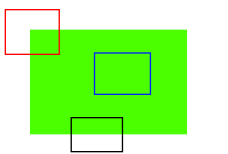## 30.Harris 角点检测

Chris_Harris 和 Mike_Stephens 早在 1988 年的文章《A Combined Corner and Edge Detector》 中就已经提出了角点检测的方法, 被称为 Harris 角点检测。它把这个简单的想法转换成了数学形式。将窗口向各个方向移动（u,v）然后计算所有差异的总和。表达式如下：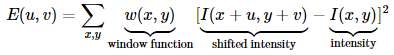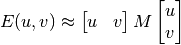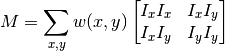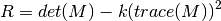• det (M ) = λ1λ2
• trace (M ) = λ1 + λ2

• λ1 和 λ2 是矩阵 M 的特征值

• 当 λ1 和 λ2 都小时,|R| 也小,这个区域就是一个平坦区域。

• 当 λ1≫ λ2 或者 λ1≪ λ2,时 R 小于 0,这个区域是边缘

• 当 λ1 和 λ2 都很大,并且 λ1～λ2 中的时,R 也很大,（λ1 和 λ2 中的最 小值都大于阈值）说明这个区域是角点。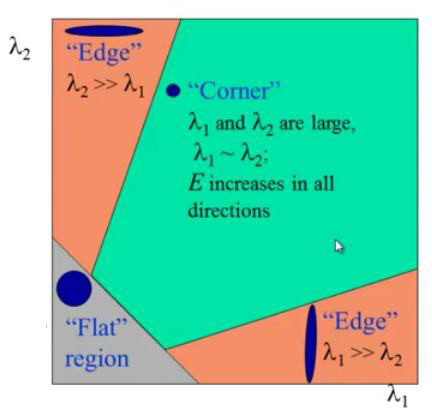### 30.1 OpenCV 中的 Harris 角点检测

OpenCV  中的函数cv2.cornerHarris() 可以用来进行角点检测。参数如下：

img - 数据类型为 ﬂoat32 的输入图像。

•  blockSize  - 角点检测中要考虑的领域大小。

ksize - Sobel 求导中使用的窗口大小

• k - Harris 角点检测方程中的自由参数,取值参数为 [0,04,0.06].

import cv2
import numpy as np

filename = 'chessboard.jpg'
gray = cv2.cvtColor(img,cv2.COLOR_BGR2GRAY)
gray = np.float32(gray)
# 输入图像必须是float32,最后一个参数在0.04 到0.05 之间
dst = cv2.cornerHarris(gray,2,3,0.04)

#result is dilated for marking the corners, not important
dst = cv2.dilate(dst,None)

# Threshold for an optimal value, it may vary depending on the image.
img[dst>0.01*dst.max()]=[0,0,255]

cv2.imshow('dst',img)
if cv2.waitKey(0) & 0xff == 27:
cv2.destroyAllWindows()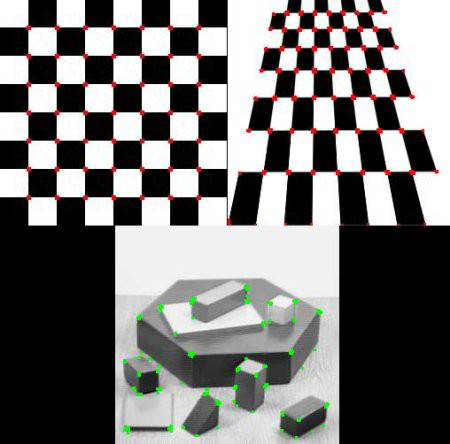### 30.2 亚像素级精确度的角点

import cv2
import numpy as np

filename = 'chessboard2.jpg'
gray = cv2.cvtColor(img,cv2.COLOR_BGR2GRAY)
# 查找Harris 角点
gray = np.float32(gray)
dst = cv2.cornerHarris(gray,2,3,0.04)
dst = cv2.dilate(dst,None)
ret, dst = cv2.threshold(dst,0.01*dst.max(),255,0)
dst = np.uint8(dst)
# 查找重心
#connectedComponentsWithStats(InputArray image, OutputArray labels, OutputArray stats,OutputArray centroids, int connectivity=8, int ltype=CV_32S)
ret, labels, stats, centroids = cv2.connectedComponentsWithStats(dst)
# 定义停止和细化角点的标准
criteria = (cv2.TERM_CRITERIA_EPS + cv2.TERM_CRITERIA_MAX_ITER, 100, 0.001)
#Python: cv2.cornerSubPix(image, corners, winSize, zeroZone, criteria)
#zeroZone – 搜索区域中间的死区大小的一半，在下面的公式中求和没有完成。它有时用来避免自相关矩阵可能的奇异性。(-1，-1)的值表示没有这样的大小.
# 返回值由角点坐标组成的一个数组（而非图像）
corners = cv2.cornerSubPix(gray,np.float32(centroids),(5,5),(-1,-1),criteria)
# Now draw them
res = np.hstack((centroids,corners))
#np.int0 可以用来省略小数点后面的数字（非四舍五入）。 res = np.int0(res) img[res[:,1],res[:,0]]=[0,0,255]
img[res[:,3],res[:,2]] = [0,255,0]

cv2.imwrite('subpixel5.png',img)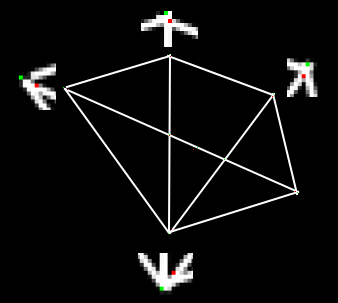## 31.Shi-Tomasi角点检测 & 适合于跟踪的图像特征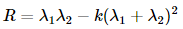R = min (λ1, λ2)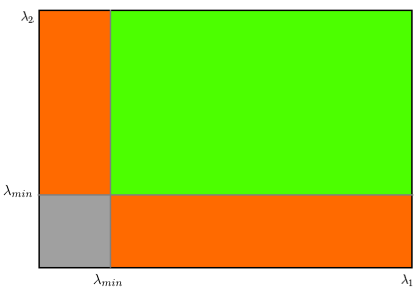OpenCV 提供了函数：cv2.goodFeaturesToTrack()。这个函数可以帮我们使用 Shi-Tomasi 方法获取图像中 N 个最好的角点（也可以通过改变参数来使用 Harris 角点检测算法）。通常情况下,输入的应该 是灰度图像。然后确定你想要检测到的角点数目。再设置角点的质量水平在0 到 1 之间。它代表了角点的最低质量,低于这个数的所有角点都会被忽略。最后在设置两个角点之间的最短欧式距离。

import numpy as np
import cv2
from matplotlib import pyplot as plt

gray = cv2.cvtColor(img,cv2.COLOR_BGR2GRAY)
corners = cv2.goodFeaturesToTrack(gray,25,0.01,10)
# 返回的结果是  [[ 311.,  250.]] 两层括号的数组。
corners = np.int0(corners)
for i in corners:
x,y = i.ravel()
cv2.circle(img,(x,y),3,255,-1)
plt.imshow(img),plt.show()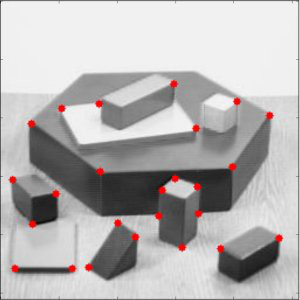## 32.SIFT(尺度不变特征变换)介绍

### 32.1原理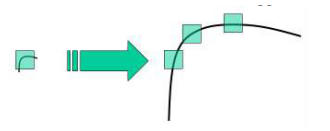(1).尺度空间极值检测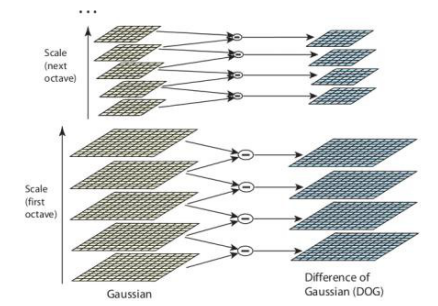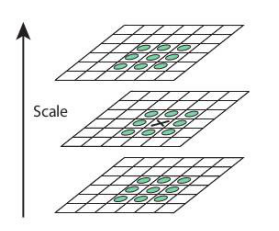(2).关键点（极值点）定位

DoG   算法对边界非常敏感,所以我们必须要把边界去除。前面我们讲的Harris 算法除了可以用于角点检测之外还可以用于检测边界。作者就是使用了同样的思路。作者使用 2x2 的 Hessian 矩阵计算主曲率。从 Harris 角点检测的算法中,我们知道当一个特征值远远大于另外一个特征值时检测到的是边界。所以它们使用了一个简单的函数,如果比例高于阈值（OpenCV 中称为边界阈值）,这个关键点就会被忽略。文章中给出的边界阈值为 10。

(3).为关键点（极值点）指定方向参数

(4).关键点描述符

(5).关键点匹配

### 32.2 OpenCV 中的 SIFT

import cv2
import numpy as np

gray= cv2.cvtColor(img,cv2.COLOR_BGR2GRAY)

sift = cv2.SIFT()
kp = sift.detect(gray,None)
img=cv2.drawKeypoints(gray,kp)
cv2.imwrite('sift_keypoints.jpg',img)

OpenCV 也提供了绘制关键点的函数：cv2.drawKeyPoints(),它可以 在关键点的部位绘制一个小圆圈。如果你设置参数为 cv2.DRAW_MATCHES_FLAGS_DRAW_RICH_ 就会绘制代表关键点大小的圆圈甚至可以绘制除关键点的方向。

img=cv2.drawKeypoints(gray,kp,flags=cv2.DRAW_MATCHES_FLAGS_DRAW_RICH_KEYPOINTS)
cv2.imwrite('sift_keypoints.jpg',img)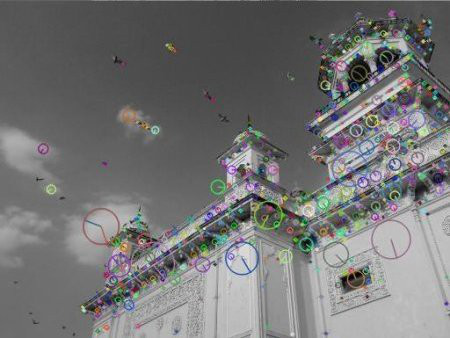(1).既然已经找到了关键字，那么可以调用sift.compute()，它根据找到的关键字计算描述符。例如:kp,des = sift.compute(gray，kp)

(2).如果没有找到关键字，可以使用函数sift.detectAndCompute()在单个步骤中直接找到关键字和描述符。

sift = cv.xfeatures2d.SIFT_create()
kp, des = sift.detectAndCompute(gray,None)

## 33.SURF(Speeded-Up Robust Features)介绍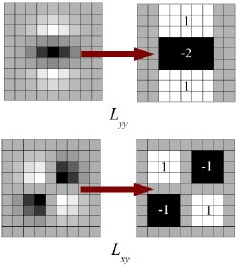SURF提供了成为U-SURF的功能,它具有更快的速度,同时保持了对+/-15度旋转的稳定性。OpenCV对这两种模式同样支持,只需要对参数upright进行设置,当upright为0时计算方向,为1时不计算方向,同时速度更快。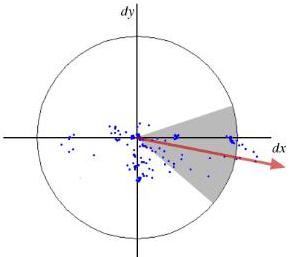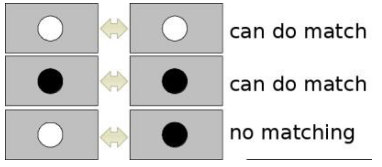OpenCV中的SURF:

img = cv.imread('fly.png',0)
# 创建SURF对象。您可以在这里或稍后指定参数。这里将Hessian阈值设置为400
surf = cv.xfeatures2d.SURF_create(400)
# 直接查找关键字和描述符
kp, des = surf.detectAndCompute(img,None)
print(len(kp))  # 699


# 检查当前Hessian阀值
print( surf.getHessianThreshold() )    # 400.0

# 我们把它定在50000左右。记住，它只是用来表示图像的。在实际情况中，值最好是300-500
surf.setHessianThreshold(50000)
# 再次计算关键点并检查它的编号.
kp, des = surf.detectAndCompute(img,None)
print( len(kp) )    # 47

img2 = cv.drawKeypoints(img,kp,None,(255,0,0),4)
plt.imshow(img2),plt.show()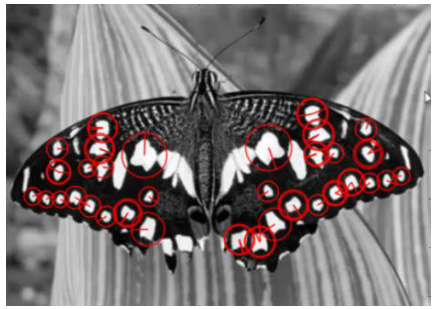# 检查upright标志，如果它是False，设置为True
print( surf.getUpright() )    #False

surf.setUpright(True)
# 重新计算特征点并绘制它
kp = surf.detect(img,None)
img2 = cv.drawKeypoints(img,kp,None,(255,0,0),4)
plt.imshow(img2),plt.show()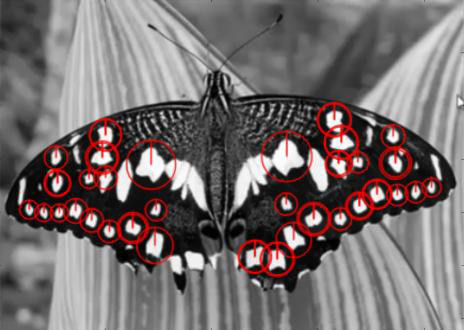# 查找描述符的大小
print( surf.descriptorSize() )    # 64
# 这意味着"extended"为False.
surf.getExtended()    # False
# 所以我们将其设置成True得到128个模糊描述符.
surf.setExtended(True)
kp, des = surf.detectAndCompute(img,None)
print( surf.descriptorSize() )    # 128
print( des.shape )    # (47, 128)


## 34.角点检测的FAST算法

### 34.1使用FAST算法进行特征提取

1.在图像中选取一个像素点p,来判断它是不是关键点。Ip等于像素点p的灰度值。

2.选择适当的阈值t。

3.如下图所示在像素点p的周围选择16个像素点进行测试。

4.如果在这16个像素点中存在n个连续像素点的灰度值都高于Ip+t,或者低于Ip−t,那么像素点p就被认为是一个角点。如上图中的虚线所示,n选取的值为12。

5.为了获得更快的效果,还采用了而外的加速办法。首先对候选点的周围每个90度的点：1,9,5,13进行测试（先测试1和19,如果它们符合阈值要求再测试5和13）。如果p是角点,那么这四个点中至少有3个要符合阈值要求。如果不是的话肯定不是角点,就放弃。对通过这步测试的点再继续进行测试（是否有12的点符合阈值要求）。这个检测器的效率很高,但是它有如下几条缺点：

•当n<12时它不会丢弃很多候选点(获得的候选点比较多)。
•像素的选取不是最优的,因为它的效果取决与要解决的问题和角点的分布情况。
•高速测试的结果被抛弃
•检测到的很多特征点都是连在一起的。

### 34.2机器学习的角点检测器

1.选择一组训练图片（最好是跟最后应用相关的图片）

2.使用FAST算法找出每幅图像的特征点

3.对每一个特征点,将其周围的16个像素存储构成一个向量。对所有图像都这样做构建一个特征向量P

4.每一个特征点的16像素点都属于下列三类中的一种。

5.根据这些像素点的分类,特征向量P也被分为3个子集：Pd,Ps,Pb

6.定义一个新的布尔变量Kp,如果p是角点就设置为Ture,如果不是就设置为False.

7.使用ID3算法（决策树分类器）查询每个子集，使用变量Kp查询关于真类的知识。它选择产生关于候选像素是否是角的最多信息的x，通过Kp的熵来测量。

8.这是递归地应用于所有的子集，直到它的熵为零。

9.将构建好的决策树运用于其它图像的快速的检测。

### 34.3 非极大值抑制

1.对所有检测到到特征点构建一个打分函数V。V就是像素点p与周围16个像素点差值的绝对值之和。

2.计算临近两个特征点的打分函数V。

3.忽略V值最低的特征点.

### 34.4 OpenCV中FAST特征检测器

cv2.FAST_FEATURE_DETECTOR_TYPE_5_8;
cv2.FAST_FEATURE_DETECTOR_TYPE_7_12 ;
cv2.FAST_FEATURE_DETECTOR_TYPE_9_16。

import numpy as np
import cv2
from matplotlib import pyplot as plt

# Initiate FAST object with default values
fast = cv2.FastFeatureDetector()
# find and draw the keypoints
kp = fast.detect(img, None)
img2 = cv2.drawKeypoints(img, kp, color=(255, 0, 0))
# Print all default params
print("Threshold: ", fast.getInt('threshold'))
print("nonmaxSuppression: ", fast.getBool('nonmaxSuppression'))
print("neighborhood: ", fast.getInt('type'))
print("Total Keypoints with nonmaxSuppression: ", len(kp))
cv2.imwrite('fast_true.png', img2)
# Disable nonmaxSuppression fast.setBool('nonmaxSuppression',0)
kp = fast.detect(img,None)
print("Total Keypoints without nonmaxSuppression: ", len(kp))
img3 = cv2.drawKeypoints(img, kp, color=(255, 0, 0))
cv2.imwrite('fast_false.png', img3)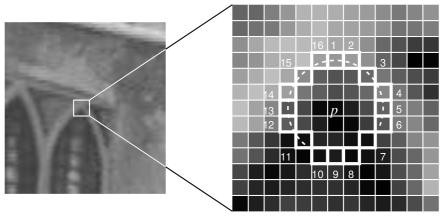FAST 算法比其它角点检测算法都快。 但是在噪声很高时不够稳定,这是由阈值决定。

## 35.BRIEF(二值稳健的独立补充特征)

BRIEF (Binary Robust Independent El-ementary Features)应运而生。它不去计算描述符而是直接找到一个二进制字符串。这 种算法使用的是已经平滑后的图像,它会按照一种特定的方式选取一组像素点 对 nd (x,y),然后在这些像素点对之间进行灰度值对比。例如,第一个点对的 灰度值分别为 p 和 q。如果 p 小于 q,结果就是 1,否则就是 0。就这样对 nd 个点对进行对比得到一个 nd  维的二进制字符串。

nd 可以是 128,256,512。OpenCV 对这些都提供了支持,但在默认 情况下是 256（OpenC 是使用字节表示它们的,所以这些值分别对应与 16, 32,64）。当我们获得这些二进制字符串之后就可以使用汉明距离对它们进行 匹配了。

OpenCV 中的 BRIEF:

import numpy as np
import cv2
from matplotlib import pyplot as plt

# Initiate STAR detector
star = cv2.FeatureDetector_create("STAR")
# Initiate BRIEF extractor
brief = cv2.DescriptorExtractor_create("BRIEF")
# find the keypoints with STAR
kp = star.detect(img, None)
# compute the descriptors with BRIEF
kp, des = brief.compute(img, kp)
print(brief.getInt('bytes'))
print(des.shape)

## 36.ORB(定向快且旋转短的BRIEF)

ORB 基本是 FAST 关键点检测和 BRIEF 关键点描述器的结合体,并通 过很多修改增强了性能。首先它使用 FAST 找到关键点,然后再使用 Harris 角点检测对这些关键点进行排序找到其中的前 N 个点。它也使用金字塔从而产 生尺度不变性特征。但是有一个问题,FAST 算法步计算方向。那旋转不变性 怎样解决呢？作者进行了如下修改。

BRIEF 有一个重要的性质，那就是每个位元特徵都有一个大的变异数和一个接近0.5的均值。但是一旦它沿着关键点方向定向，它就会失去这种特性，变得更加分散。由于对输入的响应不同，高方差使特征更具有鉴别性。另一个可取的特性是使测试不相关，因为这样每个测试都有助于结果。为了解决所有这些问题，ORB在所有可能的二进制测试中进行贪婪搜索，以找到方差和平均值都接近0.5且不相关的测试。其结果称为BRIEF。

OpenCV中的ORB算法:

import numpy as np
import cv2
from matplotlib import pyplot as plt

# Initiate STAR detector
orb = cv2.ORB()
# find the keypoints with ORB
kp = orb.detect(img, None)
# compute the descriptors with ORB
kp, des = orb.compute(img, kp)
# 只绘制关键点的位置，而不是大小和方向
img2 = cv2.drawKeypoints(img, kp, color=(0, 255, 0), flags=0)
plt.imshow(img2), plt.show()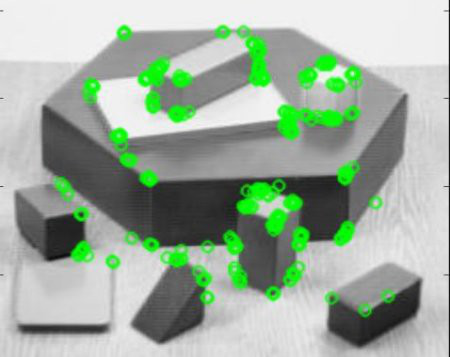## 37.特征匹配

### 37.1 Brute-Force 匹配的基础

BFMatcher 对象具有两个方法,BFMatcher.match() BFMatcher.knnMatch()。 第一个方法会返回最佳匹配。第二个方法为每个关键点返回 k  个最佳匹配（降

(1).对 ORB 描述符进行蛮力匹配

import numpy as np
import cv2
from matplotlib import pyplot as plt

img2 = cv2.imread('box_in_scene.png',0) # trainImage

# Initiate SIFT detector
orb = cv2.ORB()
# find the keypoints and descriptors with SIFT
kp1, des1 = orb.detectAndCompute(img1,None)
kp2, des2 = orb.detectAndCompute(img2,None)
# create BFMatcher object
bf = cv2.BFMatcher(cv2.NORM_HAMMING, crossCheck=True)
# Match descriptors.
matches = bf.match(des1,des2)
# Sort them in the order of their distance.
matches = sorted(matches, key = lambda x:x.distance)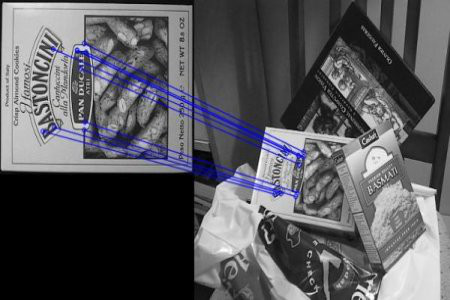(2).匹配器对象

matches = bf.match(des1, des2) 返回值是一个 DMatch 对象列表。这个DMatch 对象具有下列属性：

• DMatch.distance - 描述符之间的距离。越小越好。
• DMatch.trainIdx - 目标图像中描述符的索引。
• DMatch.imgIdx - 目标图像的索引。
• DMatch.queryIdx - 查询图像中描述符的索引。

(3).对 SIFT 描述符进行蛮力匹配和比值测试

import numpy as np
import cv2
from matplotlib import pyplot as plt

img2 = cv2.imread('box_in_scene.png', 0)  # trainImage
# Initiate SIFT detector
sift = cv2.SIFT()
# find the keypoints and descriptors with SIFT
kp1, des1 = sift.detectAndCompute(img1, None)
kp2, des2 = sift.detectAndCompute(img2, None)
# BFMatcher with default params
bf = cv2.BFMatcher()
matches = bf.knnMatch(des1, des2, k=2)
# Apply ratio test
# 比值测试,首先获取与A 距离最近的点B（最近）和C（次近）,只有当B/C小于阈值时（0.75）才被认为是匹配,因为假设匹配是一一对应的,真正的匹配的理想距离为0
good = []
for m, n in matches:
if m.distance < 0.75 * n.distance: good.append([m])

# cv2.drawMatchesKnn expects list of lists as matches.
img3 = cv2.drawMatchesKnn(img1, kp1, img2, kp2, good[:10], flags=2)
plt.imshow(img3), plt.show()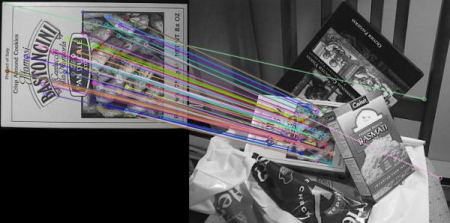### 37.2 FLANN 匹配器

FLANN 是快速最近邻搜索包（Fast_Library_for_Approximate_Nearest_Neighbors） 的简称。它是一个对大数据集和高维特征进行最近邻搜索的算法的集合,而且 这些算法都已经被优化过了。在面对大数据集时它的效果要好于 BFMatcher。 我们来对第二个例子使用   FLANN  匹配看看它的效果。

indexparams = dict(algorithm = FLANNI NDEXKDTREE, trees = 5)

import numpy as np
import cv2
from matplotlib import pyplot as plt

img2 = cv2.imread('box_in_scene.png', 0)  # trainImage
# Initiate SIFT detector
sift = cv2.SIFT()
# find the keypoints and descriptors with SIFT
kp1, des1 = sift.detectAndCompute(img1, None)
kp2, des2 = sift.detectAndCompute(img2, None)
# BFMatcher with default params
bf = cv2.BFMatcher()
matches = bf.knnMatch(des1, des2, k=2)
# Apply ratio test
good = []
for m, n in matches:
if m.distance < 0.75 * n.distance: good.append([m])

# cv2.drawMatchesKnn expects list of lists as matches.
img3 = cv2.drawMatchesKnn(img1, kp1, img2, kp2, good, flags=2)
plt.imshow(img3), plt.show()

import numpy as np
import cv2
from matplotlib import pyplot as plt

img2 = cv2.imread('box_in_scene.png', 0)  # trainImage
# Initiate SIFT detector
sift = cv2.SIFT()
# find the keypoints and descriptors with SIFT
kp1, des1 = sift.detectAndCompute(img1, None)
kp2, des2 = sift.detectAndCompute(img2, None)
# FLANN parameters
FLANN_INDEX_KDTREE = 0
index_params = dict(algorithm = FLANN_INDEX_KDTREE, trees = 5)
search_params = dict(checks=50)    # or pass empty dictionary

flann = cv2.FlannBasedMatcher(index_params,search_params)
matches = flann.knnMatch(des1,des2,k=2)
# Need to draw only good matches, so create a mask
matchesMask = [[0,0] for i in range(len(matches))]
# ratio test as per Lowe's paper
for i,(m,n) in enumerate(matches):
if m.distance < 0.7*n.distance:

draw_params = dict(matchColor = (0,255,0),singlePointColor = (255,0,0), matchesMask = matchesMask, flags = 0)
img3 = cv2.drawMatchesKnn(img1,kp1,img2,kp2,matches,None,**draw_params)
plt.imshow(img3,),plt.show()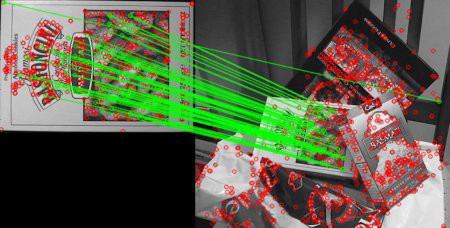38.使用特征匹配和单应性查找对象

import numpy as np
import cv2
from matplotlib import pyplot as plt

img2 = cv2.imread('box_in_scene.png', 0)  # trainImage
# Initiate SIFT detector
sift = cv2.SIFT()
# find the keypoints and descriptors with SIFT
kp1, des1 = sift.detectAndCompute(img1, None)
kp2, des2 = sift.detectAndCompute(img2, None)
FLANN_INDEX_KDTREE = 0
index_params = dict(algorithm = FLANN_INDEX_KDTREE, trees = 5)
search_params = dict(checks = 50)
flann = cv2.FlannBasedMatcher(index_params, search_params)
matches = flann.knnMatch(des1,des2,k=2)
# store all the good matches as per Lowe's ratio test.
good = []
for m,n in matches:
if m.distance < 0.7*n.distance:
good.append(m)

if len(good)>MIN_MATCH_COUNT:
# 获取关键点的坐标
src_pts = np.float32([ kp1[m.queryIdx].pt for m in good ]).reshape(-1,1,2)
dst_pts = np.float32([ kp2[m.trainIdx].pt for m in good ]).reshape(-1,1,2)
# 第三个参数  用于计算单应矩阵的方法。以下是可能的方法:
# 0 - 使用所有点的常规方法
# CV_RANSAC - RANSAC-based robust method
# CV_LMEDS - Least-Median robust method
# 第四个参数取值范围在  1 到 10, 拒绝一个点对的阈值。原图像的点经过变换后点与目标图像上对应点的误差, 超过误差就认为是  outlier
# 返回值中  M 为变换矩阵。
M, mask = cv2.findHomography(src_pts, dst_pts, cv2.RANSAC,5.0)
# 获得原图像的高和宽
h,w = img1.shape
# 使用得到的变换矩阵对原图像的四个角进行变换,获得在目标图像上对应的坐标。
pts = np.float32([ [0,0],[0,h-1],[w-1,h-1],[w-1,0] ]).reshape(-1,1,2)
dst = cv2.perspectiveTransform(pts,M)
# 原图像为灰度图
cv2.polylines(img2,[np.int32(dst)],True,255,10, cv2.LINE_AA)

else:
print ("Not enough matches are found - %d/%d" % (len(good),MIN_MATCH_COUNT))
matchesMask = None

draw_params = dict(matchColor = (0,255,0), # draw matches in green color
singlePointColor = None,
flags = 2)
img3 = cv2.drawMatches(img1,kp1,img2,kp2,good,None,**draw_params)
plt.imshow(img3, 'gray'),plt.show()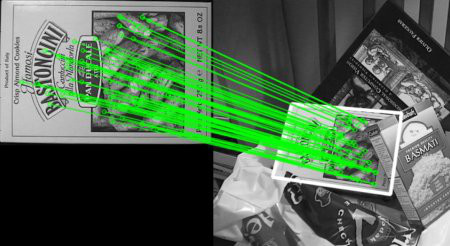# 第六章.视频分析(运动目标检测)

## 39.Meanshift 和 Camshift

### 39.1 Meanshift

Meanshift(均值偏移) 算法的基本原理很简单。假设我们有一堆点（可以是像直方图反投影那样的像素分布）和一个小窗口(可能是一个圆圈),我们要完成的任务就是将这个窗口移动到最大灰度密度处（或者是点最多的地方）。如下图所示：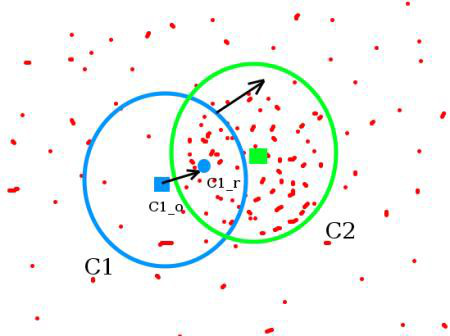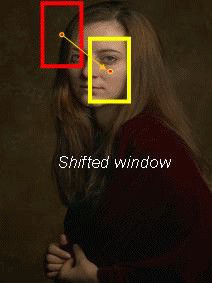import numpy as np
import cv2

cap = cv2.VideoCapture('slow.flv')
# take first frame of the video
# setup initial location of window
r,h,c,w = 250,90,400,125  # simply hardcoded the values
track_window = (c,r,w,h)
# set up the ROI for tracking
roi = frame[r:r+h, c:c+w]
hsv_roi =  cv2.cvtColor(frame, cv2.COLOR_BGR2HSV)
mask = cv2.inRange(hsv_roi, np.array((0., 60.,32.)), np.array((180.,255.,255.)))
cv2.normalize(roi_hist,roi_hist,0,255,cv2.NORM_MINMAX)
# Setup the termination criteria, either 10 iteration or move by atleast 1 pt
term_crit = ( cv2.TERM_CRITERIA_EPS | cv2.TERM_CRITERIA_COUNT, 10, 1 )

while(1):
if ret == True:
hsv = cv2.cvtColor(frame, cv2.COLOR_BGR2HSV)
dst = cv2.calcBackProject([hsv],,roi_hist,[0,180],1)
# apply meanshift to get the new location
ret, track_window = cv2.meanShift(dst, track_window, term_crit)
# Draw it on image
x,y,w,h = track_window
img2 = cv2.rectangle(frame, (x,y), (x+w,y+h), 255,2)
cv2.imshow('img2',img2)
k = cv2.waitKey(60) & 0xff
if k == 27:
break
else:
cv2.imwrite(chr(k)+".jpg",img2)
else:
break

cv2.destroyAllWindows() cap.release()### 39.2 Camshiftimport numpy as np
import cv2

cap = cv2.VideoCapture('slow.flv')
# take first frame of the video
# setup initial location of window
r,h,c,w = 250,90,400,125  # simply hardcoded the values
track_window = (c,r,w,h)
# set up the ROI for tracking
roi = frame[r:r+h, c:c+w]
hsv_roi =  cv2.cvtColor(frame, cv2.COLOR_BGR2HSV)
mask = cv2.inRange(hsv_roi, np.array((0., 60.,32.)), np.array((180.,255.,255.)))
cv2.normalize(roi_hist,roi_hist,0,255,cv2.NORM_MINMAX)
# Setup the termination criteria, either 10 iteration or move by atleast 1 pt
term_crit = ( cv2.TERM_CRITERIA_EPS | cv2.TERM_CRITERIA_COUNT, 10, 1 )
while(1):
if ret == True:
hsv = cv2.cvtColor(frame, cv2.COLOR_BGR2HSV)
dst = cv2.calcBackProject([hsv],,roi_hist,[0,180],1)
# apply meanshift to get the new location
ret, track_window = cv2.CamShift(dst, track_window, term_crit)
# Draw it on image
pts = cv2.boxPoints(ret)
pts = np.int0(pts)
img2 = cv2.polylines(frame,[pts],True, 255,2)
cv2.imshow('img2',img2)
k = cv2.waitKey(60) & 0xff
if k == 27:
break
else:
cv2.imwrite(chr(k)+".jpg",img2)
else:
break

cv2.destroyAllWindows()
cap.release()OpenCV 的官方示例中有一个 camshift 的交互式演示:

'''
Camshift tracker
================

http://www.robinhewitt.com/research/track/camshift.html
Usage:
------
camshift.py [<video source>]
To initialize tracking, select the object with mouse Keys:
-----
ESC    - exit
b  - toggle back-projected probability visualization
'''

import numpy as np
import cv2
import video    # local module

class App(object):
def   init  (self, video_src):
self.cam = video.create_capture(video_src)
cv2.namedWindow('camshift')
cv2.setMouseCallback('camshift', self.onmouse)

self.selection = None
self.drag_start = None
self.tracking_state = 0
self.show_backproj = False

def onmouse(self, event, x, y, flags, param):
x, y = np.int16([x, y])
if event == cv2.EVENT_LBUTTONDOWN:
self.drag_start = (x, y)
self.tracking_state = 0
# 官方示例中下面一行判断有问题,作如下修改就可以了
if self.drag_start and  event == cv2.EVENT_MOUSEMOVE:
#  print x,y
if flags==cv2.EVENT_FLAG_LBUTTON:
#  print 'ok'
h, w = self.frame.shape[:2]
xo, yo = self.drag_start
x0, y0 = np.maximum(0, np.minimum([xo, yo], [x, y]))
x1, y1 = np.minimum([w, h], np.maximum([xo, yo], [x, y]))
self.selection = None
if x1-x0 > 0 and y1-y0 > 0:
self.selection = (x0, y0, x1, y1)
print (self.selection)
else:
self.drag_start = None
if self.selection is not None:
self.tracking_state = 1

def show_hist(self):
bin_count = self.hist.shape

## 40.光流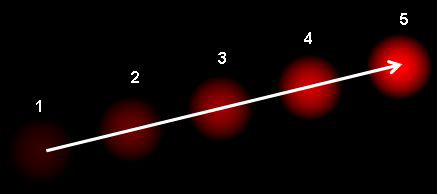• 由运动重建结构

• 视频压缩

• 视频稳定...

1.  在连续的两帧图像之间（目标对象的）像素的灰度值不改变。

2.  相邻的像素具有相同的运动

I (x, y, t) = I (x + dx, y + dy, t + dt)

fxu + fyv + ft = 0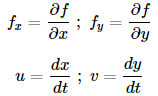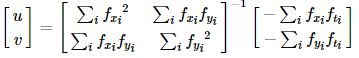（有没有发现上边的逆矩阵与 Harris 角点检测器非常相似,这说明角点很适合被用来做跟踪）

import numpy as np
import cv2

cap = cv2.VideoCapture('slow.flv')
# params for ShiTomasi corner detection
feature_params = dict(maxCorners=100, qualityLevel=0.3, minDistance=7, blockSize=7)

# Parameters for lucas kanade optical flow
# maxLevel 为使用的图像金字塔层数
lk_params = dict(winSize=(15, 15), maxLevel=2,
criteria=(cv2.TERM_CRITERIA_EPS | cv2.TERM_CRITERIA_COUNT, 10, 0.03))

# Create some random colors
color = np.random.randint(0, 255, (100, 3))
# Take first frame and find corners in it
old_gray = cv2.cvtColor(old_frame, cv2.COLOR_BGR2GRAY)
# Create a mask image for drawing purposes

while (1):
frame_gray = cv2.cvtColor(frame, cv2.COLOR_BGR2GRAY)
# calculate optical flow 能够获取点的新位置
p1, st, err = cv2.calcOpticalFlowPyrLK(old_gray, frame_gray, p0, None, **lk_params)
# Select good points
good_new = p1[st == 1]
good_old = p0[st == 1]
# draw the tracks
for i, (new, old) in enumerate(zip(good_new, good_old)):
a, b = new.ravel()
c, d = old.ravel()
frame = cv2.circle(frame, (a, b), 5, color[i].tolist(), -1)

cv2.imshow('frame', img)
k = cv2.waitKey(30) & 0xff
if k == 27:
break

# Now update the previous frame and previous points
old_gray = frame_gray.copy()
p0 = good_new.reshape(-1, 1, 2)

cv2.destroyAllWindows()
cap.release()(上面的代码没有对返回角点的正确性进行检查。图像中的一些特征点甚至 在丢失以后,光流还会找到一个预期相似的点。所以为了实现稳定的跟踪,我们应该每个一定间隔就要进行一次角点检测。OpenCV 的官方示例中带有这样 一个例子,它是每 5 帧进行一个特征点检测。它还对光流点使用反向检测来选取好的点进行跟踪。示例为/samples/python2/lk_track.py)

### 40.2 OpenCV中的稠密光流

import cv2
import numpy as np

cap = cv2.VideoCapture("vtest.avi")

prvs = cv2.cvtColor(frame1, cv2.COLOR_BGR2GRAY)
hsv = np.zeros_like(frame1)
hsv[..., 1] = 255

while (1):
next = cv2.cvtColor(frame2, cv2.COLOR_BGR2GRAY)
# cv2.calcOpticalFlowFarneback(prev, next, pyr_scale, levels, winsize, iterations, poly_n, poly_sigma, flags[)
# pyr_scale – parameter, specifying the image scale (<1) to build pyramids for each image; pyr_scale=0.5 means a classical pyramid, where each next layer is twice smaller than the previous one.
# poly_n – size of the pixel neighborhood used to find polynomial expansion in each pixel; typically poly_n =5 or 7.
# poly_sigma – standard deviation of the Gaussian that is used to smooth derivatives used as a basis for the polynomial expansion; for poly_n=5, you can set poly_sigma=1.1, for poly_n=7, a good value would be poly_sigma=1.5.
# flag(可选) - 0或1,0计算快,1慢但准确
flow = cv2.calcOpticalFlowFarneback(prvs, next, None, 0.5, 3, 15, 3, 5, 1.2, 0)

# cv2.cartToPolar Calculates the magnitude and angle of 2D vectors.
mag, ang = cv2.cartToPolar(flow[..., 0], flow[..., 1])
hsv[..., 0] = ang * 180 / np.pi / 2
hsv[..., 2] = cv2.normalize(mag, None, 0, 255, cv2.NORM_MINMAX)
rgb = cv2.cvtColor(hsv, cv2.COLOR_HSV2BGR)

cv2.imshow('frame2', rgb)
k = cv2.waitKey(30) & 0xff
if k == 27:
break
elif k == ord('s'):
cv2.imwrite('opticalfb.png', frame2)
cv2.imwrite('opticalhsv.png', rgb)
prvs = next

cap.release()
cv2.destroyAllWindows()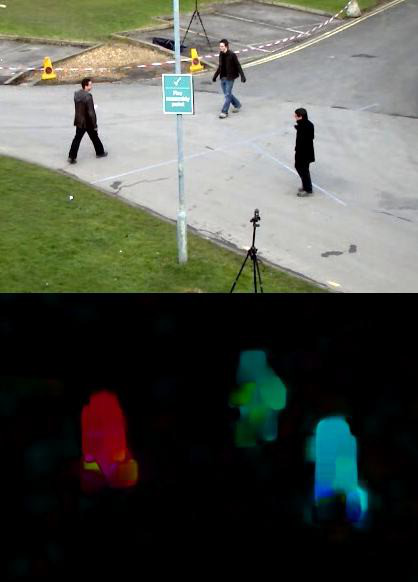OpenCV 官方示例中有一个更高级的稠密光流/samples/python2/opt_ﬂow.py！

## 41.背景减除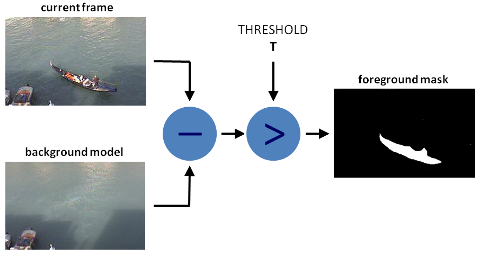1.后台初始化；

2.后台更新。

 cv::Algorithm

### (1).BackgroundSubtractorMOG

import cv2

cap = cv2.VideoCapture(0)
fgbg = cv2.createBackgroundSubtractorMOG()
while(1):
k = cv2.waitKey(30) & 0xff
if k == 27:
break
cap.release()
cv2.destroyAllWindows()MOG的结果: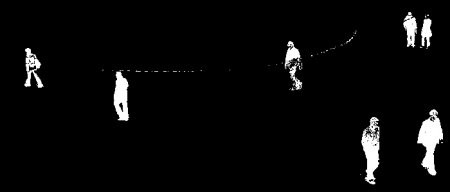### (2).BackgroundSubtractorMOG2

import cv2

cap = cv2.VideoCapture('vtest.avi')
fgbg = cv2.createBackgroundSubtractorMOG2()
while(1):
k = cv2.waitKey(30) & 0xff
if k == 27:
break
cap.release()
cv2.destroyAllWindows()

MOG2的结果(灰色区域代表阴影):### (3).BackgroundSubtractorGMG

import cv2

cap = cv2.VideoCapture('vtest.avi')
kernel = cv2.getStructuringElement(cv2.MORPH_ELLIPSE,(3,3))
fgbg = cv2.createBackgroundSubtractorGMG()
while(1):
k = cv2.waitKey(30) & 0xff
if k == 27:
break
cap.release()
cv2.destroyAllWindows()

GMG 的结果(使用形态学开运算将噪音去除):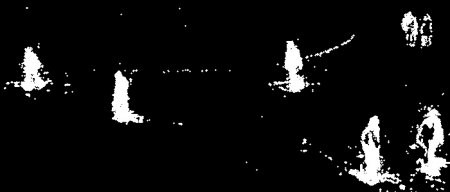### (4).BackgroundSubtractorKNN

backgroundSubtractor = cv2.createBackgroundSubtractorKNN(detectShadows=True)
structuringElement = cv2.getStructuringElement(cv2.MORPH_ELLIPSE, (3, 3))

# 利用帧差法获取矩形框
def getBoxes(frame, backgroundSubtractor, structuringElement):
boxes = []
gray_L = cv2.cvtColor(frame, cv2.COLOR_BGR2GRAY)
# 二值化阈值处理，前景掩码含有前景的白色值以及阴影的灰色值，在阈值化图像中，将非纯白色（244~255）的所有像素都设为0，而不是255
th = cv2.threshold(fgmask.copy(), 244, 255, cv2.THRESH_BINARY)
dilated = cv2.dilate(th, structuringElement, iterations=2)  # 形态学膨胀
# 该函数计算一幅图像中目标的轮廓
contours, hierarchy = cv2.findContours(image=dilated, mode=cv2.RETR_EXTERNAL,method=cv2.CHAIN_APPROX_SIMPLE)
for cnt in contours:
if cv2.contourArea(cnt) > 2000:
(x, y, w, h) = cv2.boundingRect(cnt)  # 外接矩形
boxes.append((x, y, w, h))

return frame, boxes

KNN的结果(灰色区域为检测到的阴影):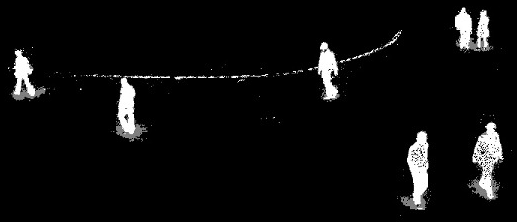01-158933
04-29
10-271102
01-29913
06-23692
06-188647
02-169814
07-08659
04-221万+
02-281万+
03-181777
03-311万+

### “相关推荐”对你有帮助么？

•非常没帮助
•没帮助
•一般
•有帮助
•非常有帮助Thomson617

¥2 ¥4 ¥6 ¥10 ¥20余额支付 (余额：-- )扫码支付获取中扫码支付点击重新获取扫码支付1.余额是钱包充值的虚拟货币，按照1:1的比例进行支付金额的抵扣。
2.余额无法直接购买下载，可以购买VIP、C币套餐、付费专栏及课程。余额充值